# 前言

HashMap是Java程序员使用最多的数据结构之一，同时也是面试必问的知识点，随着JDK的进化与发展，JDK 1.8也对底层实现进行了优化，例如引入红黑树的数据结构和扩容的优化等。本文将结合JDK 1.7和1.8的源码，深入探讨HashMap的结构实现和功能原理，篇幅有些长请耐心看完。

# 简介

HashMap和ArrayList一样也是继承一个实现一个，继承关系几乎一致，只是把List换成了Map。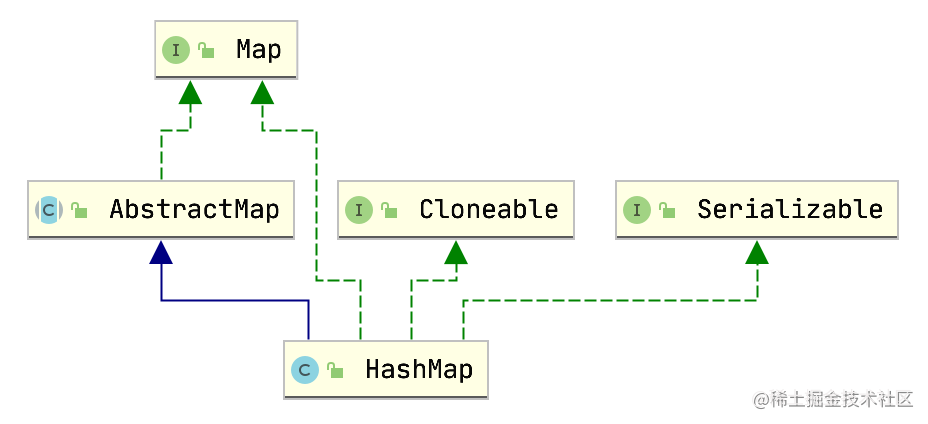# 基本原理

## 基本数据结构

HashMap的基本数据结构是数组 + 链表/红黑树，小规模情况下以数组 + 链表（类似于桶存储）为主，大量数据的时候会转换为红黑树，这里需要提的是何时会转换为红黑树，这是一个我们必须要关注的问题，会在后面讲到。

## put原理

``````// 扰动函数
static final int hash(Object key) {
int h;
return (key == null) ? 0 : (h = key.hashCode()) ^ (h >>> 16);
}

• HashMap支持key为null存储，为null的时候返回为0。
• 如果直接拿散列值作为下标访问HashMap主数组的话，考虑到2进制32位带符号的int表值范围从-2147483648到2147483648，很难出现碰撞，不过这个hash值不能直接拿来用，因为hashmap的初始值才16
• 如何处理hash值：在这之前会有一个函数叫做indexFor去处理，主要是结合长度进行模运算。在JDK8中这一操作被放到了putVal中直接处理。

``````public V put(K key, V value) {
return putVal(hash(key), key, value, false, true);
}

putVal源码注释解析

``````final V putVal(int hash, K key, V value, boolean onlyIfAbsent,
boolean evict) {
Node<K,V>[] tab; Node<K,V> p; int n, i;
// 如果table为空或者0则执行resize，并将长度赋值给n，做必要的初始化
if ((tab = table) == null || (n = tab.length) == 0)
n = (tab = resize()).length;
if ((p = tab[i = (n - 1) & hash]) == null) // 此处 & 代替了 % （除法散列法进行散列）
// 如果hash出来的值为空，则新建一个结点并插入到指定位置（意思就是tab[i]的位置此时还没有数据插入）
tab[i] = newNode(hash, key, value, null);
// 这里的p结点是根据hash值算出来对应在数组中的元素，如果为空就代表还未插入。
else {
Node<K,V> e; K k;
// 如果插入的key相同，进行覆盖操作
if (p.hash == hash &&
((k = p.key) == key || (key != null && key.equals(k))))
e = p;
// 如果是红黑树结点则进行红黑树操作
else if (p instanceof TreeNode)
e = ((TreeNode<K,V>)p).putTreeVal(this, tab, hash, key, value);
else {
// 链表操作
for (int binCount = 0; ; ++binCount) {
if ((e = p.next) == null) {
p.next = newNode(hash, key, value, null);
if (binCount >=TREEIFY_THRESHOLD- 1)// -1 for 1st
// 将链表转换成红黑树。
treeifyBin(tab, hash);
break;
}
if (e.hash == hash &&
((k = e.key) == key || (key != null && key.equals(k))))
break;
// 及时更新p
p = e;
}
}
// 如果e存在，就进行覆盖
if (e != null) {// existing mapping for key
V oldValue = e.value;
// 判断是否允许覆盖，并且oldValue此时的状态
if (!onlyIfAbsent || oldValue == null)
e.value = value;
return oldValue;
}
}
++modCount;   // 更改操作次数
// 如果容量超标，则执行resize进行扩容
if (++size > threshold)
resize();
// 结束Node插入之后的操作
afterNodeInsertion(evict);
return null;
}

1）初始化并准备好结点。

2）判断需要进行的操作，进行创建赋值（相同key，红黑树结点，链表操作）。

3）将创建玩的结点应用到Map中去。

4）容量检测。

1）在数组中如何存储对应的结点数据：通过(n - 1) & hash计算出位置再放入的。

2）链表在什么时候会转换为红黑树：binCount 大于7的时候就会转换为红黑树，当其小于6的时候就会变会链表。

## get原理

``````public V get(Object key) {
Node<K,V> e;
return (e = getNode(hash(key), key)) == null ? null : e.value;
}

getNode返回一个数的数据结点，如果为空则返回null；

``````final Node<K,V> getNode(int hash, Object key) {
Node<K,V>[] tab; Node<K,V> first, e; int n; K k;
if ((tab = table) != null && (n = tab.length) > 0 &&
(first = tab[(n - 1) & hash]) != null) {
if (first.hash == hash &&// always check first node
((k = first.key) == key || (key != null && key.equals(k))))
return first;
if ((e = first.next) != null) {
if (first instanceof TreeNode)
return ((TreeNode<K,V>)first).getTreeNode(hash, key);
// 链表的迭代
do {
if (e.hash == hash &&
((k = e.key) == key || (key != null && key.equals(k))))
return e;
} while ((e = e.next) != null);
}
}
return null;
}

1）通过 hash & (table.length - 1)获取该key对应的数据节点的hash槽。

2）判断首个结点是否为空，如果为空就直接返回null。

3）判断首个结点的hash是否与待查找的hash值相同，如果相同直接返回取出。

4）进入链表迭代的循环，流程图如下所示。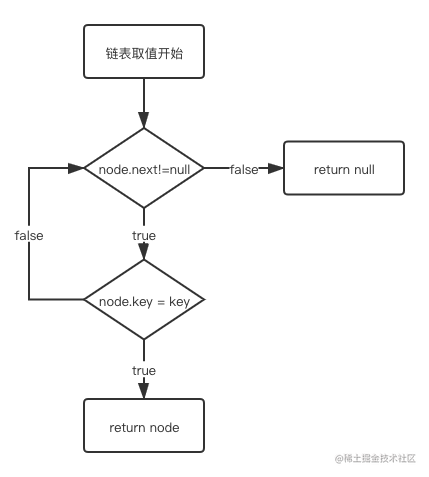``````final TreeNode<K,V> find(int h, Object k, Class<?> kc) {
TreeNode<K,V> p = this;
do {
int ph, dir; K pk;
TreeNode<K,V> pl = p.left, pr = p.right, q;
if ((ph = p.hash) > h)
p = pl;
else if (ph < h)
p = pr;
else if ((pk = p.key) == k || (k != null && k.equals(pk)))
return p;
else if (pl == null)
p = pr;
else if (pr == null)
p = pl;
else if ((kc != null ||
(kc =comparableClassFor(k)) != null) &&
(dir =compareComparables(kc, k, pk)) != 0)
p = (dir < 0) ? pl : pr;
else if ((q = pr.find(h, k, kc)) != null)
return q;
else
p = pl;
} while (p != null);
return null;
}

## Hash碰撞

HashMap使用链表来解决碰撞问题，当碰撞发生了，对象将会存储在链表的下一个节点中。hashMap在每个链表节点存储键值对对象。当两个不同的键却有相同的hashCode时，他们会存储在同一个bucket位置的链表中

## 红黑树

R-B Tree，全称是Red-Black Tree，又称为“红黑树”，它一种特殊的二叉查找树。红黑树的每个节点上都有存储位表示节点的颜色，可以是红(Red)或黑(Black)。

（1）每个节点或者是黑色，或者是红色。

（2）根节点是黑色。

（3）每个叶子节点（NIL）是黑色。[注意：这里叶子结点，是只为空的叶子结点！]

（4）如果一个节点是红色的，则它的子节点必须是黑色的。

（5）从一个节点到该节点的子孙节点的所有路径上包含相同数目的黑结点。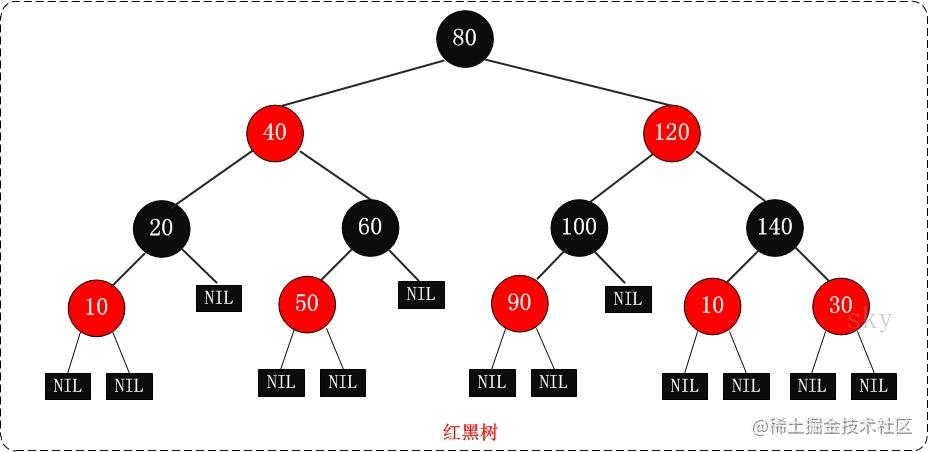### 链表到红黑树的转换

``````// 链表转双向链表操作
final void treeifyBin(Node<K,V>[] tab, int hash) {
int n, index; Node<K,V> e;
// 如果元素总个数小于64，则继续进行扩容，结点指向调节
if (tab == null || (n = tab.length) <MIN_TREEIFY_CAPACITY)
resize();
// 先找到那个链表的头
else if ((e = tab[index = (n - 1) & hash]) != null) {
TreeNode<K,V> hd = null, tl = null; // hd：树头部
do {
// 创建红黑树根结点
TreeNode<K,V> p = replacementTreeNode(e, null);
if (tl == null)
hd = p;
else {
p.prev = tl;
tl.next = p;
}
tl = p;
} while ((e = e.next) != null);
if ((tab[index] = hd) != null)
// 此处才是真正的转为红黑树（树化其实就是红黑树的创建，这里就不过多分析了）
hd.treeify(tab);
}
}

1）当结点数量为空，或少于64个的时候会自动触发resize()。

2）递归构建TreeNode，构建完成调用treeify完成树化。

## 扩容机制

``````final Node<K,V>[] resize() {
// 获得旧元素数组的各种信息
Node<K,V>[] oldTab = table;
// 获取旧容量长度
int oldCap = (oldTab == null) ? 0 : oldTab.length;
// 扩容的临界点
int oldThr = threshold;
// 定义新数组的长度，以及扩容的临界值
int newCap, newThr = 0;
// 如果旧的容量 > 0 也就是原有table不为空。
if (oldCap > 0) {
// 如果数组长度达到最大值，则修改临界值为Integer.MAX_VALUE
if (oldCap >=MAXIMUM_CAPACITY) {
threshold = Integer.MAX_VALUE;
return oldTab;
}
// 下面就是扩容操作（2倍）
else if ((newCap = oldCap << 1) <MAXIMUM_CAPACITY&&
oldCap >=DEFAULT_INITIAL_CAPACITY)
// 阈值也变为二倍
newThr = oldThr << 1;
}
else if (oldThr > 0)// 旧阈值倍设置为新容量
newCap = oldThr;
else { // 如果是新的初始化，则cap设置为默认容量
newCap =DEFAULT_INITIAL_CAPACITY;
// 如果阈值设置为默认阈值
}
if (newThr == 0) { // 如果临界值为0，则设置临界值
float ft = (float)newCap * loadFactor;
newThr = (newCap <MAXIMUM_CAPACITY&& ft < (float)MAXIMUM_CAPACITY?
(int)ft : Integer.MAX_VALUE);
}
threshold = newThr; // 更新填充因子
@SuppressWarnings({"rawtypes","unchecked"})
Node<K,V>[] newTab = (Node<K,V>[])new Node[newCap];
table = newTab;
// 下面就是扩容之后，要针对性调整红黑树或链表的指向。
if (oldTab != null) {
for (int j = 0; j < oldCap; ++j) {
Node<K,V> e;
if ((e = oldTab[j]) != null) {
oldTab[j] = null;
if (e.next == null)
newTab[e.hash & (newCap - 1)] = e;
else if (e instanceof TreeNode) // 红黑树调整
((TreeNode<K,V>)e).split(this, newTab, j, oldCap);
else {
// 链表调整
Node<K,V> loHead = null, loTail = null;
Node<K,V> hiHead = null, hiTail = null;
Node<K,V> next;
do {
next = e.next;
if ((e.hash & oldCap) == 0) {
if (loTail == null)
else
loTail.next = e;
loTail = e;
}
else {
if (hiTail == null)
else
hiTail.next = e;
hiTail = e;
}
} while ((e = next) != null);
if (loTail != null) {
loTail.next = null;
}
if (hiTail != null) {
hiTail.next = null;
}
}
}
}
}
return newTab; // 最后返回新的table data；
}

Capacity：当前HashMap的长度

• 扩容新建一个新的数组，长度为原来的两倍（这一点在源码注释上有提到）。
• 把原数组，重新Hash过后放入新的数组中去。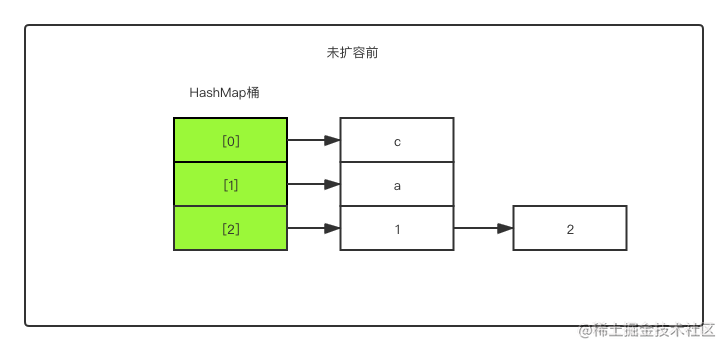未扩容前，有一个容量为3的HashMap存储桶，此时其中的数据如下所示，此时需要对HashMap进行扩容。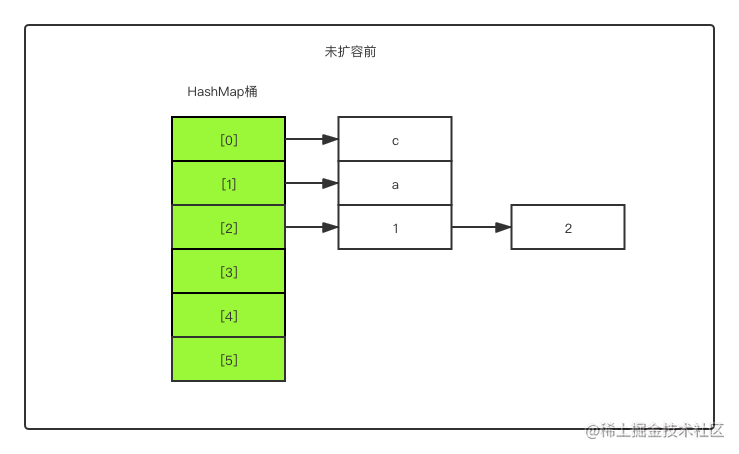如果未进行rehash，那么此时的位置如上图所示，而此时因为是已经扩容完成的，那么getNode那边在获取到对应HashMap的size将是6，而不是原来的3，那么试想一下(6 - 1) & hash 等于 (3 - 1) & hash么？答案显而易见是不等的，那么resize过后如果不重新resize，所有的以前的Node都拿不到了。

# 总结

HashMap这样的知识点还有很多，一篇文章是说不完的，所以我并没有介绍一些HashMap的基本知识，将更多的篇幅放在了一些比较关键部分的介绍，至于更多的玄机需要我们一起去探索。

# 参考文献

blog.csdn.net/AJ1101/arti…

www.zhihu.com/question/20…

blog.csdn.net/cmyperson/a…

www.cnblogs.com/skywang1234…

yuanrengu.com/2020/ba1842…

blog.csdn.net/AJ1101/arti…

www.cnblogs.com/aobing/p/12…